## Yet another practice M403K 2nd midterm from another instructor:

1) (10 points) How much money should we invest today at a rate of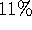compounded continuously if we need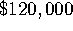in three years time?

2) (10 points) Find the differential of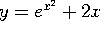when x=0 and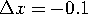.

3) (25 points) Let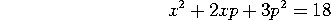be a price-demand equation for a certain product. Find the rate of change of p when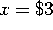and x is decreasing at a rate of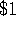per month. Is the price increasing or decreasing?

4) (55 points) Let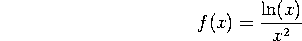We want to find the absolute maximum of f(x) on its domain. For doing that, complete the following steps.

a) (5 points) Find the domain of f.

b) (5 points) Find all intercepts.

c) (10 points) Find horizontal and vertical asymptotes, if any.

d) (20 points) Find the intervals where f is increasing and those where it is decreasing. Identify all local maxima and all local minima, if any.

e) (10 points) Graph y=f(x). You may need some of the following: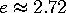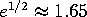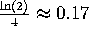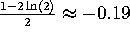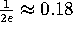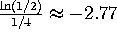f) (5 points) Identify the absolute maximum and the absolute minimum, if they exist.